# Bihar Board 12th Chemistry Model Question Paper 4 in English Medium

Bihar Board 12th Chemistry Model Papers

## Bihar Board 12th Chemistry Model Question Paper 4 in English Medium

Time : 3 Hours 15 Min
Full Marks: 70

Instructions for the candidates

1. Candidates are required to give answers in their own words as far as practicable.
2. Figures in the right hand margin indicates full marks.
3. While answering the questions, candidate should adhere to the words limit as far as practicable.
4. 15 Minutes of extra time has been allotted for the candidates to read the questions carefully.
5. This question paper is divided into two sections : Section -A and Section-B
6. In Section A, there are 35 objective type questions which are compulsory, each carrying 1 mark. Darken the circle with blue/black ball pen against the correct option on OMR Sheet provided to you. Do not use Whitener/Liquid/Blade/ Nail on OMR Sheet otherwise your result will be treated as invalid.
7. In section-B, there are 18 short answer type questions (each carrying 2 marks), out of which only 10 (ten) questions are to be answered Apart from this there are 06 Long Answer type questions (each carrying 5 marks), out of which 3 questions are to be answered.
8. Use of any electronic device is prohibited.

Objective Type Questions

In the following questions no. from 1 to 35, there is only one correct answer against each question. For each question, mark (darken) the correct answer on the OMR Sheet provided to you. (1 x 35 = 35)

Question 1.
Which type of crystals contains more than one Bravais lattice ?
(a) Hexagonal
(b) Triclinic
(c) Rhombohedral
(d) Monoclinic
(d) Monoclinic

Question 2.
For the structure given below the site marked as S is a …………….(a) tetrahedral void
(b) cubic void
(c) octahedral void
(d) none of these
(c) octahedral void

Question 3.
If the radius of an octahedral void is r and radius of atoms in close packing is R, the relation between r and R is
(a) r = 0.414 R
(b) R = 0.414 r
(c) r = 2R
(d) r = $$\sqrt{2} R$$
(a) r = 0.414 RQuestion 4.
A metal crystallises into a lattice containing a sequence of layers as AB AB AB………………… What percentage of voids are left in the lattice ?
(a) 72%
(b) 48%
(c) 26%
(d) 32%
(c) 26%

Question 5.
In ccp arrangement the pattern of successive layers can be designated as
(a) AB AB AB
(b) ABC ABC ABC
(c) AB ABC AB
(d) ABA ABA ABA
(b) ABC ABC ABC

Question 6.
A solution is obtained by mixing 200 g of 30% and 300 g of 20% solution by weight. What is the percentage of solute in the final solution ?
(a) 50%
(b) 28%
(c) 64%
(d) 24%
(d) 24%

Question 7.
When 1.04 g of BaCl2 is present in 105 g of solution the concentration of solution is ………….
(a) 104 ppm
(b) 10.4 ppm
(c) 0104 ppm
(d) 104 ppm
(b) 10.4 ppmQuestion 8.
In the cell, |Zn2+| |Cu2+|Cu, the negative terminals
(a) Cu
(b)Cu2+
(c) Zn
(d) Zn2+
(d) Zn2+

Question 9.
What will be standard cell potential of galvanic cell with the following reaction ?(a) 74 V
(b) 1.14 V
(c) 34 V
(d) – 0.34 V
(c) 34 V                         .

Question 10.
In a reaction 2HI → H2 + I2 the concentration of HI decreases from 0.5 mol L-1 in 10 minutes. What is the rate of reaction during this interval ?
(a) 5 x 10-3 M min-1
(b) 5 x 10-3 M min-1
(c) 5 x 10-2 M min-1
(d) 5 x 10-2 M min-1
(a) 5 x 10-3 M min-1

Question 11.
The rate of disappearance of SO, in the reaction, 2SO2 + O2  → 2SO3 is 1.28 x 10-5 Ms . The rate of appearance of SO3 is ……………….
(a) 64 x 10-5 M s-1
(b) 0.32 x 10-5 M s-1
(c) 56 x 10-5 Ms1
(d) 1.28 x 10-5  M s-1
(d) 1.28 x 10-5  M s-1

Question 12.
Which of the following is a property of physisorption ?
(a) High specificity
(b) Irreversibility
(c) Non-specificity
(d) None of these
(c) Non-specificityQuestion 13.
Which of the following statement is not correct about physisorption ?
(a) It is a reversible process
(b) It requires less heat of adsorption
(c) It requires activation energy
(d) It takes place at low temperature
(c) It requires activation energy

Question 14.
The name of the metal which is extracted from the ore is given below. Mark the example which is not correct.
(a) Malachite – Cu
(b) Calamine – Zn
(c) Chromite – Cr
(d) Dolomite – Al
(d) Dolomite – Al

Question 15.
Which of the followings is magnetite ?
(a) Fe2CO3
(b) Fe2O3
(c) Fe3O4
(d) Fe2O3.3H2O
(c) Fe3O4

Question 16.
Nitrogen can form only one chloride with chlorine which is NCl3 whereas P can form PCl3 and PCl5. This is ……………
(a) due to absence of d-orbitals in nitrogen
(b) due to difference in size of N and P
(c) due to higher reactivity of P towards Cl than N
(d) due to presence of multiple bonding in nitrogen
(a) due to absence of d-orbitals in nitrogenQuestion 17.
On heating a mixture of NH4Cl and KNO2, we get
(a) NH4NO3
(b) KNH4(NO3)2
(c) N2
(d) NO
(c) N2

Question 18.
The melting point of copper is higher than that of zinc because
(a) the s, p as well as d-electrons of copper are involved in metallic bonding
(b) the atomic volume of copper is higher
(c) the d-electrons of copper are involved in metallic bonding
(d) the s as well as d-electrons of copper are involved in metallic bonding
(c) the d-electrons of copper are involved in metallic bonding

Question 19.
Zr and Hf have almost equal atomic and ionic radii because of ………………….
(a) diagonal relationship
(b) lanthanoid contraction
(c) actinoid contraction
(d) belonging to the same group
(b) lanthanoid contraction

Question 20.
Which of the following primary and secondary valencies are not correctly marked against the compounds ?
(a) [Cr(NH3)6]Cl3, p = 3,s = 6
(b) K2[Pt(CI4],p = 2,s = 4
(c) [Pt(NH3)2Cl2],p = 2,s = 4
(d) [Cu(NH3)4]SO4,p = 4,s = 4
(d) [Cu(NH3)4]SO4,p = 4,s = 4

Question 21.
When aqueous solution of potassium fluoride is added to the blue coloured aqueous CuSO4 solution, a green precipitate is formed. This observation can be explained as follows.
(a) On adding KF, H2O being weak field ligand is replaced by F ions forming [CuF4 ]2- which is green in colour
(b) Potassium is coordinated to [Cu(H2O)4]2+ ion present in CuSO4 and gives green colour
(c) On adding KF, Cu2+ are replaced by K+ forming a green complex
(d) Blue colour of CuSO4 and yellow colour of KI form green colour on mixingAnswer:
(a) On adding KF, H2O being weak field ligand is replaced by F ions forming [CuF4 ]2- which is green in colour

Question 22.
IUPAC name of CH3)2 CH — CH2 — CH2Br is
(a) 1-bromopentane
(b) 1-bromo-3-methylbutane
(c) 2-methyl-4-bromobutane
(d) 2-methyl-3-bromopropane
(b) 1-bromo-3-methylbutaneQuestion 23.
The IUPAC name of the compound is(a) 1 -fluoro-4-methyl-2-nitrobenzene
(b) 4-fIu6ro-lmethyl-3-nitrobenzene
(c) 4-methyl-l-fIuoro-2-nitrobenzene
(d) 2-fIuoro-5-methyl-l-nitrobenzene
(d) 2-fIuoro-5-methyl-l-nitrobenzene

Question 24.
The C— O — H angle in ether is about
(a) 180°
(b) 190°28′
(c) 110°
(d) 105°
(c) 110°

Question 25.
The C— O — H bond angle in alcohols is slightly less than the tetrahedral angle whereas the C— O — C bond angle in ether is slightly greater because ………
(a) of repulsion between the two bulky R groups.
(b) O atom in both alcohols and ethers is sp3-hybridised
(c) lone pair-lone pair repulsion is greater than bond pair­bond pair repulsion
(d) none of these
(a) of repulsion between the two bulky R groups.

Question 26.
Propanone can be prepared from ethyne by
(a) passing a mixture of ethyne and steam over a catalyst, magnesium at 420°C
(b) passing a mixture of ethyne and ethanol over a catalyst zinc chromite
(c) boiling ethyne with water and H2SO4
(d) treating ethyne with iodine and NaOH
(a) passing a mixture of ethyne and steam over a catalyst, magnesium at 420°C

Question 27.
In the following reaction, product (P) is(a) RCHO
(b) RCH3
(c) RCOOH
(d) RCH2OH
(a) RCHOQuestion 28.
IUPAC name of the compound (C2H5)2 NCH is
(a) 2, 2-diethylmethanamine
(b) N, N-diethylmethanamine
(c) N-ethyl-N-methylethanamine
(d) N-methylbutanamine
(c) N-ethyl-N-methylethanamine

Question 29.
Reduction of aromatic nitro compounds using Sn and HCI gives
(a) armatic primary amines
(b) aromatic secondary amines
(c) aromatic tertiary amines
(d) aromatic amides
(a) armatic primary amines

Question 30.
Which of the fol lowing is an example of an aldopentose ?
(a) D-Ribose
(b) Glyceraldehyde
(c) Fructose
(d) Erythrose
(a) D-Ribose

Question 31.
Which of the following treatment will convert starch directly into glucose ?
(a) Heating with dilute H2SO4
(b) Fermentation by diastase
(c) Fermentation by zymase
(d) Heating with dilute NaOH
(a) Heating with dilute H2SO4

Question 32.
The correct structure of monomers of buna-S is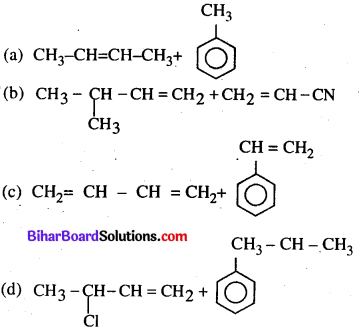(c)

Question 33.
The S in buna-S refers to
(a) sulphur
(b) styrene
(c) sodium
(d) salicylate
(b) styreneQuestion 34.
The main cause of acidity in the stomach is
(a) release of extra gastric acid which decrease the pH level
(b) indidestion and pain in large intestine
(c) increase the pH level in the stomach
(d) release of extra bile juice which increase alkaline medium in stomach.
(a) release of extra gastric acid which decrease the pH level

Question 35.
Which of the following will not act as antacid ?
(a) Sodium hydrogen carbonate
(b) Magnesium hydroxide
(c) Sodium carbonate
(d) Aluminium carbonate
(c) Sodium carbonate

Non-Objective Type Questions

In this Section, there are 18 Short answer type questions (each carrying 2 marks), out of which answer any 10 questions.  2 x 10 = 20

Question 1.
Calculate the osmotic pressure of 5% solution of urea at 272 K . (R = 0.0821 L-atm. deg-1).
As we know that $$\pi=\frac{n_{\text {urea }} R T}{V}$$
Number of moles in urea $$=\frac{5}{60}=0.083$$
Volume of Solution $$=\frac{100}{1000}=0.1$$ litres
Hence, Osmotic Pressure, $$\pi=\frac{0.083 \times 0.0821 \times 272}{0.1}=18.53 \mathrm{atm}$$
Osmotic Pressure = 18.53atm

Question 2.
Define standard electrode potential.
Standard Electrode Potential : The standard electrode potential is the potential difference between the electrode & electrolyte (at 1 M), at standard conditions (1 atm, 298 K). Standard electrode potential is abbreviated by (E or E°).

Question 3.
What is activation energy ? How is the rate constant of a reaction related to its activation energy ?
Activation Energy: The minimum extra amount of energy absorbed by the reactant molecules so that their energy becomes equal to threshold value is called activation energy.
Activation energy (Ea)= Threshold energy (ET) – Average energy of the reactant (ER). i.e. Ea = ET – ER
If activation energy will be less, then faster will be the reaction.
i. e . Activation energy = $$\alpha \frac{1}{\text { rate of reaction }}$$
e.g. 2NO + O2 → 2NO2
It is a fast reaction because of low activation energy. 2CO + O2 → 2CO2
It is a slow reaction because of high activation energy.

Question 4.
What is the main difference between physiorption and Chemisorption ?
Physisorption:

• Force of attraction are Vander Waal’s forces.
• It usually takes place at low temperature and decreases with increasing temperature.
• It does not require any activation energy.

Chemisorption :

• Force of attraction are chemical bond forces.
• It takes place at high temperature.
• It requires high activation energy.Question 5.
Differentiate the following giving suitable example:
(i) Calcination and Roasting
(ii) Flux and Slag.
(i) Calcination :
(a) Calcination is a thermal treatment process in absence of air applied to ores and materials to bring about a thermal decomposition, phase transition or removal of volalatile fraction It is done at temperature below the melting point of the product material.
(b)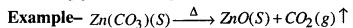Roasting:
(a) Roasting is reverse process of calcination. It is also thermal process in presence of air applied to ore for removal of volatile fraction under melting point of the product material is called Roasting.
(b)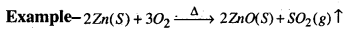Flux :
(a) It is chemical cleaning agent, which remove impurities from metal.
(b) Example – CaO + SiO2 → CaSiO3

Slag :
(a) Slag is partially vitereous by product of the process of smelting ores, which separates the desiredmetal fraction from the unwanted fraction. It is mixture of metal oxide
(b) Example – CaO + SiO2 → CaSiO3

Question 6.
Why do transition elements form coloured compounds ? Explain.
The value of electrods potential depends upon the heat of sublimation and ionisation energy
ΔH – ΔHsub + IE + ΔHhyd . Due to the influence of ligand d-orbitals of transition metals divide into two unequal energy containing sets. Unpaired electrons absorb sunlight and jumps from one orbit into another orbit and one clour is reflected.

Question 7.
Predict the geometrical shapes of the following:
(a) sp3
(b)d2sp3
(a) sp3 tetra-hedral
(b)d2SP3 – Square tetrahedral.

Question 8.
Specify oxidation numbers of the metals in the following co-ordination compounds:
(a) K4[Fe(CN)6]
(b) [PtCl4]2-
(a) Oxidation number of (co-ordination compound)
Let oxidation number of Fe = x
Hence, 1 x 3 + (x -1 x 6 = 0
or, x – 3 = 0
x = 3 = O. N of Fe

(b) Oxidation number of (co-oridnation compaund)
Hence, x + 4 (-1) = – 2
or, x = -2 + 4 = + 2 = ON of PtQuestion 9.
Write the structural formulae of the following:
(a) 4,4 dimethyl-2-pentanol
(b) 2-butanol.
(a) 4, 4 dimethyl, 2-Pentanol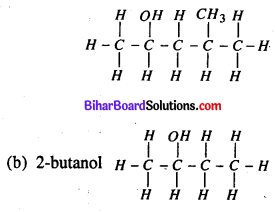Question 10.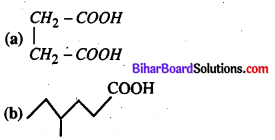Question 11.
How will you convert the following ?
(a) Ethyl alcohol to ethylamine
(b) Ethylamine to ethyl alcohol.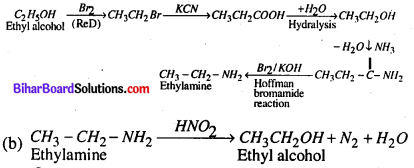Question 12.
Explain in which of the following compounds, the chemical bond would have less ionic character: LiCl or KCl.
LiCl > KCl – Due to large size of k, KCl compound has less ionic character. LiCl is covalent than Li+ has higher polarisation capacity.

Question 13.
Discuss briefly the structure of CsCl.
Structure of CsCl.Question 14.
The osmotic pressure of sugar solution is 2.46 atm at 27°C. Calculate the concentration of the solution.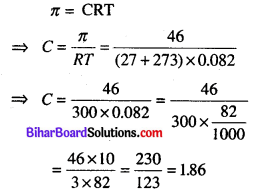Question 15.
How is molarity of a solution different from molality?
Molarity : It is defined as the number of moles of solute dissolved in one liter of solution
$$Molarity (\mathrm{M})=\frac{\text { Moles of solute }}{\text { Volume of Solution in liter }}$$

Molality : It is defined as the number of moles of solute dissolved in one kg of solvent
$$Molality (\mathrm{m})=\frac{\text { Moles of the Solute }}{\text { Mass of the solvent in } \mathrm{kg}}$$Question 16.
Define heat of neutralisation.
Defination of heat of neutralization : The heat reaction resulting from the neutralization of an acid or base especially the quantity produced when a gram equivalent of a base or acid is neutralized with a gram equivalent of an acid or base in dilute soliution.

Question 17.
(a) State law of mass action.
(b) What is the effect of temperature on reaction ?
(a) Law of mass action: Law stating that the rate of any chemical reaction is proportional to the product of the masses of the reacting substances, with each mass raised to a power equal to the coefficient that occurs in the chemical equation.

(b) The effect of increasing collision frequency on the rate of the reaction is very minor increasing the temperature increases reaction rates because of the disproportionately large increase in the number of high energy collisions.

Question 18.
Discuss the following terms :
(a) Coordination number
(b) Effective atomic number.
(a) The coordination number of a central atom in a molecure or crystal is the number of its near neighbours. This number is determined some what differently for molecules than for crystals.

(b) Effective atomic number has two different meaning; one that is the effective nuclear charge of an atom, and other that calculates the average atomic number for a compound or mixture of materials.

There are 06 long answer type questions (each carrying 05 marks), out of which answer any there questions.
(5 x 3 = 15)

Question 19.
Define order to reaction and molecularity of relation. Derive a general expression for specific rate constant of first order reaction.
It is the numbers of atom, ion, and molecule that collide with one another simultaneously so as to result into a chemical reaction
Rate constant → It is the speed at which the reactions are converted into the products at any moment of time.
Law of Mass Action → At a given temperature, the rate of a chemical reaction is directly proportional to the product of molar concentrations of reacting species with each concentration term raised to the power equal to numerical co-efficient of that species in the chemical reaction.
Thus, A + B → Products
Rate of reaction, r x [A] [B] = K [A] [B]
Where, [A] and [B] are the molar concentrations of the reactants A and B respectively and K is a constant of proportionality.
∴Rate = K [A]a [B]b

First Order Reaction – It is the type of reaction in which the rate of reaction depends only on the first power of the concentration of a single reacting Species.
Let, A + B → Product
If, a be the initial concentration of A and after time t, the concentration of product is x, then the concentration of A left at that instant is (a – x)
A → Products
initial conc.n (a)  ….(i)
after time ‘t’ (a – x)  … (ii)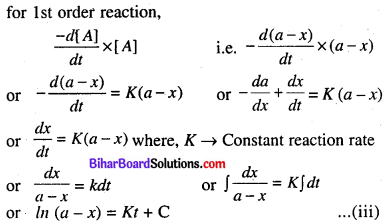Where, C → integration constant, which can be obtained from initial condition of the reaction, when, t = 0, x = 0,
then, from (iii) In (a – 0) = K x 0 + C
or, C = In (a)  ………….. (iv)
from (iii) + (iv) – In (a -x) = Kt – In (a)
or, In (a) – In (a – x) = kt or, In (a) – In (a – x) = kt.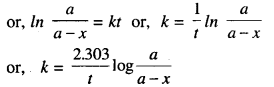Question 20.
(a) Differentiate between DNA and RNA.
(b) What are Nucleic acids ?
(a) The structural difference between DNA and RNA
DNA : DNA is double stranded helix in which two strands are coiled spirally in opposite directions.

• The sugar molecule is 2-dehydrobase.
• Nitrogeneous base uracil is not present.
• DNA molecule are very large their molecules weights may vary from 6 million to 16.
• DNA has unique property of replication.

RNA :

• It is single strainded structure.
• The sugar molecules is ribose.
• Nitrogeneous base they-mine is not present.
• RNA molecules are much smaller with molecular weight ranging from 20000 to 40000.
• RNA usually doesn’t replicate.

(b) Nucleic acid – Nucleic acid are found in all living cells in form of nucleo proteins. They constitute important class of biomolecules. Nucleic acids are genetic material of the cells and are responsible for transmission of herediatry effect from one generation to the other and also carry out the biosynthesis of proteins. Nucleic acid are biopolymers (i.e., polymers present in living system). The genetic information about in nucleic acid controls the structure of all proteins including enzymes and thus governs the entire metabolic activity in the living organism.

Question 21.
(a) Discuss the principle involved in the manufacture of sulphuric acid by contact process.
(b) How will you test for sulphate ion ?
From following steps sulphuric acid is manufactured
(i) SO2 gas is produced by burning sulphur of FeS2 in excess of air
S + O2  → SO2
4 FeS2 +11O2 → 2Fe2 O3 + 8SO2

(ii) SO2 is oxidised into SO3 in prensence of catalyst
2 SO2 + O2 → 2 SO3

(iii) SO3 on treatment with cone. H2SO4 oleum (H2S2O7) is obtained.
SO3 + H2SO4  → 2 H2S22O7

(iv) From oleum sulphuric acid is obtained of desired concentration by diluting water.
H2S2O7+ H2O → 2H2OSO4

(b) Test of sulphate radical (of NO2SO4) – We mix up Barium chloride in the salt. We get white precipate of BaSO4 which is insoluble in dil. HCl & HNO3.
BaCl2 4- Na2SO4 → BaSO4 ↓ +2 NaCl
BaCl2 + HNO3 → In solubleQuestion 22.
What are carbohydrates ? How are they classified ?
Carbohydrates are the naturally occuring organic compounds and are a major source of energy to our body. In plants carbohydrates are formed as a result for photosynthesis.In animal systems the carbohydrates undergo decomposition to form carbon-dioxide and water accompanied by the release of energy needed for the body.

Composition of carbohydrates – The chemical formula of the carbohydrates suggests that these are the hydrates of carbon. For example, glucose may be represented as C6(H2O)6 while sucrose as C12(H2O)11 . But this definition has certain limitation.

(i) Compounds like formaldehyde (CH2O) and acetic acid (C2H4O2) are the hydrates of carbon do not show characteristics of carbohydrates.

(ii) Similarly, compound like rhammose (C6H12O6) and deoxyribose (C5H10O4) are carbohydrates but not the hydrates of carbon.

The definition of carbohydrates has been modified and they may be defined as—The polyhydroxy aldehydes or polyhdroxy ketones are substances which generally give these on hydrolysis; contain atleast one chiral carbon and are therefore, opitcally active classification of carbohydrates—

Classification of carbohydrates are done in three way

(a) Based on molecular size – on the basis of the molecular size, carbohydrates have been classified into three types. These are

• Manosaccharides
• Oligosoccharides
• Polysaccharides

Based on taste – Carbohydrates with sweet taste are called sugar while those without a sweet taste are called non-sugars. It may be noted that all mono and oligosaccharides are sugars while polysaccharides are non­sugar.

Reducing and non-reducing sugar –  Carbohydrate which reduce Tollen’s reagent and Fehling solution are called reducing sugar while those which don’t reduce, these are called non reducing sugars. For example – glucose and fructose.

Question 23.
Disuss the following :
(a) Kolbe’s reaction
(b) Wurtz’s reaction
(c) Carbylamine reaction.
(a) Kolbe’s reaction – The reaction of phenoxide ion with CO2 at the high pressure and temperature followed by acidification to form salicylic acid is called Kolbe’s reaction.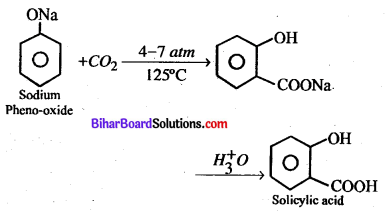(b) Wurtz reaction-When two moles of alkyl halide is heated with Na in the presence of ether goes higher alkane.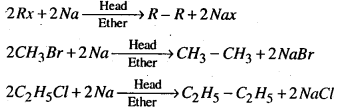(c) Carbylamine reaction-Chloroform when heated with primary amine in presence of alcoholic KOH forms a derivative called isocyanide.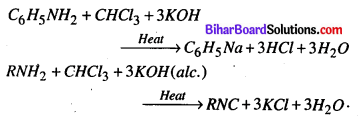Question 24.
Give one example for each of the following :
(a) Synthetic rubber
(b) Naturally occurring amino acid
(c) Condensation polymer